# Tennis & Data Science¶

Short description:
Tennis & Data science is a Foundley project with Mladen Jovanović as a mentor. The main goal of this project is data exploration, prepocessing, feature extraction and visualization. Mladen provided a dataset "03_tenisai_data.csv" which contains information about the tennis match between Novak Đoković and Rodger Federer from Wimbledon men's single finals in 2015.
In following cells reader can get to know with the results that I found interesting, statistic of the match in general and code used to process data and visualize it.

# Importing necessary libraries¶

In :
import numpy as np
import matplotlib.pyplot as plt
import pandas as pd
import seaborn as sn


In :
df = pd.read_csv('03_tennisai_data.csv')


# Dataset preview¶

In :
print("Dimensions of the dataset: ", df.shape)

Dimensions of the dataset:  (422, 52)

Out:
match_id frame_pos p1 p1_sets p1_games p1_points p1_set1 p1_set2 p1_set3 p1_set4 ... lob rally_length rally_1-4 rally_5-8 rally_9+ expected_ballhit_count detected_ballhit_count game_id point_description strokes_timestamps
0 3 350 djokovic 0 0 0 -1 -1 -1 -1 ... 0 1 1 0 0 1 1 0 1st serve wide, ace. [26.192]
1 3 975 djokovic 0 0 0 -1 -1 -1 -1 ... 0 2 1 0 0 3 3 0 1st serve wide, fault (net). 2nd serve to body... [41.239, 49.412, 50.341]
2 3 1425 djokovic 0 0 0 -1 -1 -1 -1 ... 0 3 1 0 0 3 3 0 1st serve wide; forehand return down the middl... [65.945, 66.688, 67.802]
3 3 1850 djokovic 0 0 15 -1 -1 -1 -1 ... 0 1 1 0 0 1 2 0 1st serve down the T, ace. [85.264, 86.378]
4 3 2275 djokovic 0 0 15 -1 -1 -1 -1 ... 0 3 1 0 0 4 4 0 1st serve wide, fault (net). 2nd serve down th... [101.053, 109.97, 110.899, 112.385]

5 rows × 52 columns

The dataset is structured from 422 rows representing each point played in matches, and 52 columns for storing information about players' names, point winner, strokes, current game, set, point, etc. Every row also contains point description columns that includes point flow with detailed description of that specific point.

# Check for NaNs¶

In :
# Check for NaN values in dataset
print("There is%s NaNs in dataset" %(" not" if not df.isnull().values.any() else ""))

There is not NaNs in dataset


# Match result¶

In :
# Evaluate final result for every set
p1_set_list = [df['p1_set'+str(i)].iloc[-1] for i in range(1,6) if df['p1_set'+str(i)].iloc[-1] != -1]
p2_set_list = [df['p2_set'+str(i)].iloc[-1] for i in range(1,6) if df['p2_set'+str(i)].iloc[-1] != -1]

# Evaluate set score for final set
if(df['p1_sets'].iloc[-1] == df['p2_sets'].iloc[-1]):
if(df['matchpoint'].iloc[-1]==1):
p1_set_list.append(df['p1_games'].iloc[-1]+1)
p2_set_list.append(df['p2_games'].iloc[-1])
elif(df['matchpoint'].iloc[-1]==2):
p1_set_list.append(df['p1_games'].iloc[-1])
p2_set_list.append(df['p2_games'].iloc[-1]+1)
else:
raise ValueError("There is no winner!")

# Match winner
player1_name = df['p1'].iloc.upper() + df['p1'].iloc[1::]
player2_name = df['p2'].iloc.upper() + df['p2'].iloc[1::]
player_name = [player1_name, player2_name]
match_winner = player1_name if(p1_set_list > p2_set_list) else player2_name

# Dictionary for match result data
match_result = {}

for num_set in range(len(p1_set_list)):
match_result['Set ' + str(num_set+1)] = [p1_set_list[num_set], p2_set_list[num_set]]

if(match_winner == player1_name):
match_result['Winner'] = [True, False]
else:
match_result['Winner'] = [False, True]

match_result_df = pd.DataFrame(data=match_result)
match_result_df.index = [player1_name, player2_name]

Out:
Set 1 Set 2 Set 3 Set 4 Set 5 Winner
Djokovic 7 1 7 4 13 True
Federer 6 6 6 6 12 False

Columns for sets and games for players 1 and 2 was used to track result to final row, where 'matchpoint' column was used to determine a winner.

# Serve analysis¶

## Overall serve analysis¶

In :
fig, axes = plt.subplots(nrows=1, ncols=2, figsize=(14,6), facecolor='white')
ax = axes.ravel()
plt.suptitle('Service overall', fontweight='bold')

for i,p in zip(range(2),range(1,3)):
# Points by serve
serve_df = df.loc[(df['server']==player_name[i].lower())]

# Number of services
N_serve = serve_df.shape

# Aces
N_aces = serve_df.loc[serve_df['ace'] == p].shape

# Double faults
N_double_f = serve_df.loc[serve_df['double_fault'] == p].shape
# Single faults
N_single_f = serve_df.loc[serve_df['2nd_serve'] == p].shape

values = [
N_aces,
N_single_f,
N_double_f,
serve_df.shape - (N_aces+N_single_f+N_double_f)
]
names = [
'Aces',
'Single faults',
'Double faults',
'Other'
]

ax[i].pie(values,
labels=names,
labeldistance=1.15,
wedgeprops = { 'linewidth' : 3, 'edgecolor' : 'white' },
autopct = '%.2f%%')
ax[i].set_title(player_name[i])

plt.show()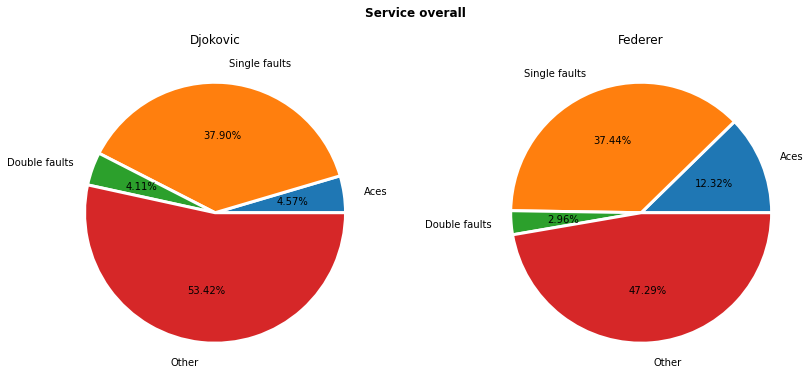From the pie chart shown above, we can conclude that Federer is much more confident in his serve having more aces (almost three times) and less double faults (almost double) among all his serves comparing to Đoković.

## Service by sets¶

In :
# Number of sets
N_sets = len(p1_set_list)

# List for important data
aces_set = []
first_fault_set = []
second_fault_set = []
points_won_ratio = []
point_duration = []

for i,p in zip(range(2),range(1,3)):
# Points by serve
serve_df = df.loc[(df['server']==player_name[i].lower())]

aces_set_ = []
first_fault_set_ = []
second_fault_set_ = []
points_won_ratio_ = []
point_duration_ = []

for s in range(1, N_sets+1):
# Current set
if(s == 1):
set_df = serve_df.loc[serve_df['p'+str(p)+'_set'+str(s)] == -1]
else:
set_df = serve_df.loc[(serve_df['p'+str(p)+'_set'+str(s)] == -1) &
(serve_df['p'+str(p)+'_set'+str(s-1)] != -1)]

# Aces in current set
N_ace = set_df.loc[set_df['ace'] == p].shape

# Double faults
N_double_fault = set_df.loc[set_df['double_fault'] == p].shape
# Single faults
N_single_fault = set_df.loc[set_df['2nd_serve'] == p].shape

# Points won and lost in first serve
N_won_ratio = 100*set_df.loc[(set_df['pt_won_by'] == p)].shape/set_df.shape

# Append values to list
aces_set_.append(N_ace)
first_fault_set_.append(N_single_fault)
second_fault_set_.append(N_double_fault)
points_won_ratio_.append(N_won_ratio)

# Average point duration
point_duration_.append(np.mean(set_df['point_duration']))

aces_set.append(aces_set_)
first_fault_set.append(first_fault_set_)
second_fault_set.append(second_fault_set_)
points_won_ratio.append(points_won_ratio_)
point_duration.append(point_duration_)

# x axis
sets = np.arange(1, N_sets+1)
set_names = tuple(['Set '+str(s) for s in sets])

# Aces per sets
plt.figure(figsize=(12,6))
plt.bar(sets-0.1, aces_set, width=0.2, color='r', align='center')
plt.bar(sets+0.1, aces_set, width=0.2, color='g', align='center')
plt.ylabel('Number of aces')
plt.xticks(sets, set_names)
plt.title('Aces per set')
plt.legend(player_name)
plt.grid('minor')
plt.show()

# Faults per sets
plt.figure(figsize=(12,6))
plt.bar(sets-0.15, first_fault_set, width=0.1, color='y', align='center')
plt.bar(sets-0.05, first_fault_set, width=0.1, color='r', align='center')
plt.bar(sets+0.05, second_fault_set, width=0.1, color='g', align='center')
plt.bar(sets+0.15, second_fault_set, width=0.1, color='b', align='center')
plt.xticks(sets, set_names)
plt.ylabel('Service faults')
plt.title('Service faults per set')
plt.legend([player_name+'\'s single fault', player_name+'\'s single fault',
player_name+'\'s double fault', player_name+'\'s double fault'])
plt.grid('minor')
plt.show()

# Percentage of points won per set
plt.figure(figsize=(12,6))
plt.bar(sets-0.1, points_won_ratio, width=0.2, color='m', align='center')
plt.bar(sets+0.1, points_won_ratio, width=0.2, color='c', align='center')
plt.ylabel('Points won by server [%]')
plt.xticks(sets, set_names)
plt.ylim([0, 100])
plt.title('Server\'s points won per set')
plt.legend(player_name)
plt.grid('minor')
plt.show()

# Average point duration per set
plt.figure(figsize=(12,6))
plt.bar(sets-0.1, point_duration, width=0.2, color='g', align='center')
plt.bar(sets+0.1, point_duration, width=0.2, color='y', align='center')
plt.ylabel('Average point duration [s]')
plt.xticks(sets, set_names)
plt.title('Average winning point duration per set')
plt.legend(player_name)
plt.grid('minor')
plt.show()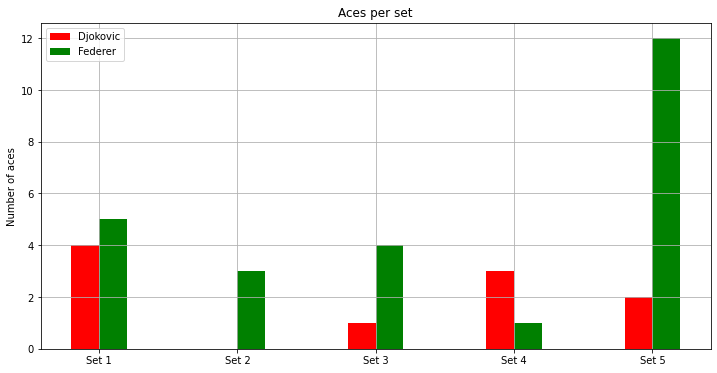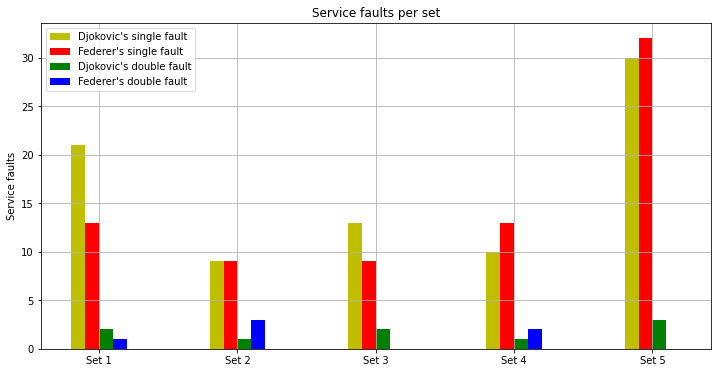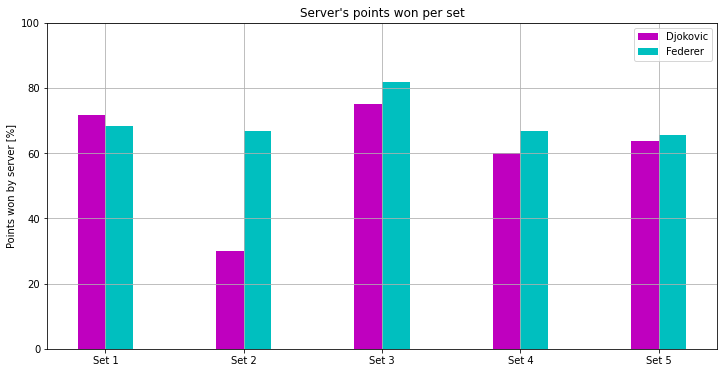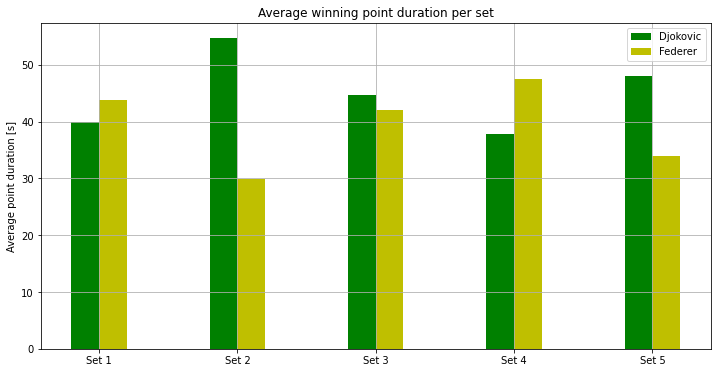Number Đoković's aces seem to drop during the match slightly more aggressive on average. Federer dominated in last set scoring 6 times more aces than his opponent. Interestingly, from number of aces and the number of faults in each set per player, Federer appears to have lost sets in which he had much better service than Đoković (more aces and less faults). Looking at the percent of points won by a server and average point duration of points on each players' service, every set, except second, players has played pretty balanced comparing to each other. The only one different is the second set in which Đoković lost 70% of the points in his service, but with with average point duration of nearly a minute, so result of this set (1-6) didn't actually mean that Đoković "gave up" on this site.

## First and second service¶

In :
fs_pts = []
ss_pts = []

for i,p in zip(range(2),range(1,3)):
# Points by serve
serve_df = df.loc[df['server']==player_name[i].lower()]

# First service data
fs_df = serve_df.loc[serve_df['2nd_serve']==0]

# Second service data
ss_df = serve_df.loc[serve_df['2nd_serve']==p]

# First service won
Nfs_won = fs_df.loc[fs_df['pt_won_by'] == p].shape
# First service lost
Nfs_lost = fs_df.shape - Nfs_won
fs_pts.append([Nfs_won, Nfs_lost])

# Second service won
Nss_won = ss_df.loc[ss_df['pt_won_by'] == p].shape
# Second service lost
Nss_lost = ss_df.shape - Nss_won
ss_pts.append([Nss_won, Nss_lost])

fig, axes = plt.subplots(nrows=2, ncols=2, figsize=(8,9), dpi=120, facecolor='white')
ax = axes.ravel()
plt.suptitle('Points won in first and second service', fontweight='bold')

for i in range(2):
ax[i*2].pie([fs_pts[i], fs_pts[i]],
labels=['Server', 'Opponent'],
labeldistance=1.15,
wedgeprops = { 'linewidth' : 3, 'edgecolor' : 'white' },
autopct = '%.2f%%')
ax[i*2].set_title(player_name[i]+'\'s first serve')

ax[i*2+1].pie([ss_pts[i], ss_pts[i]],
labels=['Server', 'Opponent'],
labeldistance=1.15,
wedgeprops = { 'linewidth' : 3, 'edgecolor' : 'white' },
autopct = '%.2f%%')
ax[i*2+1].set_title(player_name[i]+'\'s second serve')

plt.show()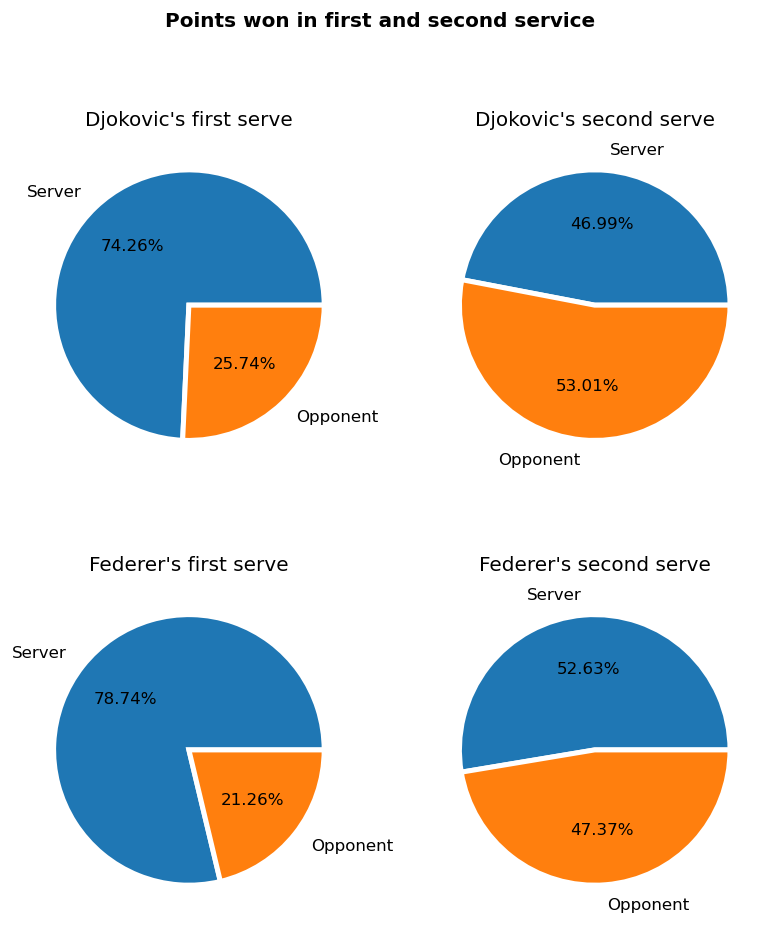Service number turned out to have a great influence of point outcome. Both players won around 75% of points on their first service, but only around 50% when the first service was an error. Đoković even has fewer points won on his second service than his opponent.

## Players service type and direction analysis¶

Having a point description column in dataset helps going through all types of services played in the match. In the following cells, all types of service, including faults, are explored.

In :
# Player 1
serve_df = df.loc[df['server']==player_name.lower()]

fs_df1 = pd.DataFrame(0,
columns=['ace', 'service winner', 'fault (long)', 'fault (wide)', 'fault (net)', 'fault (wide and long)', 'regular'],
index = ['wide', 'down the T', 'to body'],
dtype = int)
fs_df1.index = ['wide', 'down the T', 'to body']

ss_df1 = pd.DataFrame(0,
columns=['ace', 'service winner', 'fault (long)', 'fault (wide)', 'fault (net)', 'fault (wide and long)', 'regular'],
index = ['wide', 'down the T', 'to body'],
dtype = int)
ss_df1.index = ['wide', 'down the T', 'to body']

for i in range(serve_df.shape):
serve_desc = serve_df['point_description'].iloc[i].split(';').split('.')

# Categorize first serve
for desc in serve_desc:
if('1st' in desc):
# Remove string '1st serve'
desc_list = desc[10::].split(',')

if(len(desc_list) == 1):
fs_df1.loc[desc_list, 'regular'] += 1
else:
fs_df1.loc[desc_list, desc_list[1::]] += 1

elif('2nd' in desc):
# Remove string ' 2nd serve'
desc_list = desc[11::].split(',')

if(len(desc_list) == 1):
ss_df1.loc[desc_list, 'regular'] += 1
else:
ss_df1.loc[desc_list, desc_list[1::]] += 1

# Player 2
serve_df = df.loc[df['server']==player_name.lower()]

fs_df2 = pd.DataFrame(0,
columns=['ace', 'service winner', 'fault (long)', 'fault (wide)', 'fault (net)', 'fault (wide and long)', 'regular'],
index = ['wide', 'down the T', 'to body'],
dtype = int)
fs_df2.index = ['wide', 'down the T', 'to body']

ss_df2 = pd.DataFrame(0,
columns=['ace', 'service winner', 'fault (long)', 'fault (wide)', 'fault (net)', 'fault (wide and long)', 'regular'],
index = ['wide', 'down the T', 'to body'],
dtype = int)
ss_df2.index = ['wide', 'down the T', 'to body']

for i in range(serve_df.shape):
serve_desc = serve_df['point_description'].iloc[i].split(';').split('.')

# Categorize first serve
for desc in serve_desc:
if('1st' in desc):
# Remove string '1st serve'
desc_list = desc[10::].split(',')

if(len(desc_list) == 1):
fs_df2.loc[desc_list, 'regular'] += 1
else:
fs_df2.loc[desc_list, desc_list[1::]] += 1

elif('2nd' in desc):
# Remove string ' 2nd serve'
desc_list = desc[11::].split(',')

if(len(desc_list) == 1):
ss_df2.loc[desc_list, 'regular'] += 1
else:
ss_df2.loc[desc_list, desc_list[1::]] += 1


### First player's first service stats¶

In :
fs_df1.head()

Out:
ace service winner fault (long) fault (wide) fault (net) fault (wide and long) regular
wide 4 2 10 9 21 3 52
down the T 5 0 9 4 16 1 53
to body 0 0 6 0 4 0 20

### First player's second service stats¶

In :
ss_df1.head()

Out:
ace service winner fault (long) fault (wide) fault (net) fault (wide and long) regular
wide 0 0 1 1 1 0 22
down the T 1 0 0 0 1 0 19
to body 0 0 4 0 1 0 32

### Second player's first service stats¶

In :
fs_df2.head()

Out:
ace service winner fault (long) fault (wide) fault (net) fault (wide and long) regular
wide 10 1 4 18 18 4 59
down the T 15 0 11 9 11 0 40
to body 0 0 0 0 1 0 2

### Second player's second service stats¶

In :
ss_df2.head()

Out:
ace service winner fault (long) fault (wide) fault (net) fault (wide and long) regular
wide 0 0 0 0 1 0 32
down the T 0 0 4 0 0 0 17
to body 0 0 0 0 1 0 21

## Service direction¶

In :
wide = [fs_df1.loc['wide'].to_numpy().sum() + ss_df1.loc['wide'].to_numpy().sum(),
fs_df2.loc['wide'].to_numpy().sum() + ss_df2.loc['wide'].to_numpy().sum()]

down_the_T = [fs_df1.loc['down the T'].to_numpy().sum() + ss_df1.loc['down the T'].to_numpy().sum(),
fs_df2.loc['down the T'].to_numpy().sum() + ss_df2.loc['down the T'].to_numpy().sum()]
to_body = [fs_df1.loc['to body'].to_numpy().sum() + ss_df1.loc['to body'].to_numpy().sum(),
fs_df2.loc['to body'].to_numpy().sum() + ss_df2.loc['to body'].to_numpy().sum()]

fig, axes = plt.subplots(nrows=1, ncols=2, figsize=(14,6), facecolor='white')
ax = axes.ravel()
plt.suptitle('Service direction', fontweight='bold')

for i in range(2):
values = [wide[i], down_the_T[i], to_body[i]]
names = ['Wide','Down the T','To body']

ax[i].pie(values,
labels=names,
labeldistance=1.15,
wedgeprops = { 'linewidth' : 3, 'edgecolor' : 'white' },
autopct = '%.2f%%')
ax[i].set_title(player_name[i])
plt.show()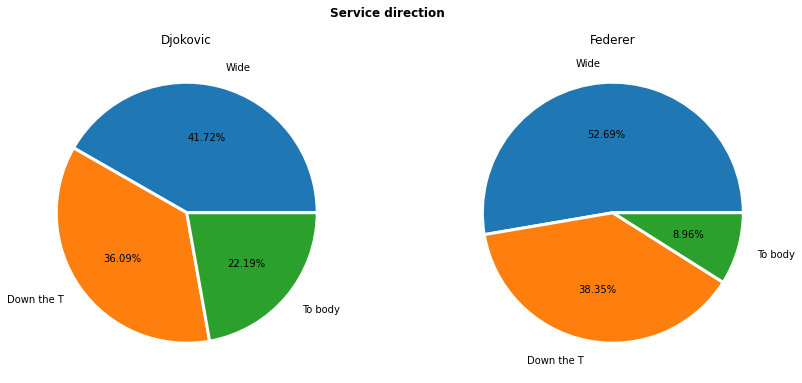Both Đoković and Federer prefer wide services over other types with Federer having nearly three times less services to body then Đoković.

### First and second service direction¶

In :
# Player 1
wide = [fs_df1.loc['wide'].to_numpy().sum(), ss_df1.loc['wide'].to_numpy().sum()]
down_the_T = [fs_df1.loc['down the T'].to_numpy().sum(), ss_df1.loc['down the T'].to_numpy().sum()]
to_body = [fs_df1.loc['to body'].to_numpy().sum(), ss_df1.loc['to body'].to_numpy().sum()]

fig, axes = plt.subplots(nrows=1, ncols=2, figsize=(14,6), facecolor='white')
ax = axes.ravel()
plt.suptitle(player_name + '\'s service direction (first & second)', fontweight='bold')
for i in range(2):
values = [wide[i], down_the_T[i], to_body[i]]
names = ['Wide','Down the T','To body']
ax[i].pie(values,
labels=names,
labeldistance=1.15,
wedgeprops = { 'linewidth' : 3, 'edgecolor' : 'white' },
autopct = '%.2f%%')
if(i==0):
ax[i].set_title('First service')
else:
ax[i].set_title('Second service')
plt.show()

# Player 2
wide = [fs_df2.loc['wide'].to_numpy().sum(), ss_df2.loc['wide'].to_numpy().sum()]
down_the_T = [fs_df2.loc['down the T'].to_numpy().sum(), ss_df2.loc['down the T'].to_numpy().sum()]
to_body = [fs_df2.loc['to body'].to_numpy().sum(), ss_df2.loc['to body'].to_numpy().sum()]

fig, axes = plt.subplots(nrows=1, ncols=2, figsize=(14,6), facecolor='white')
ax = axes.ravel()
plt.suptitle(player_name + '\'s service direction (first & second service)', fontweight='bold')
for i in range(2):
values = [wide[i], down_the_T[i], to_body[i]]
names = ['Wide','Down the T','To body']
ax[i].pie(values,
labels=names,
labeldistance=1.15,
wedgeprops = { 'linewidth' : 3, 'edgecolor' : 'white' },
autopct = '%.2f%%')
if(i==0):
ax[i].set_title('First service')
else:
ax[i].set_title('Second service')
plt.show()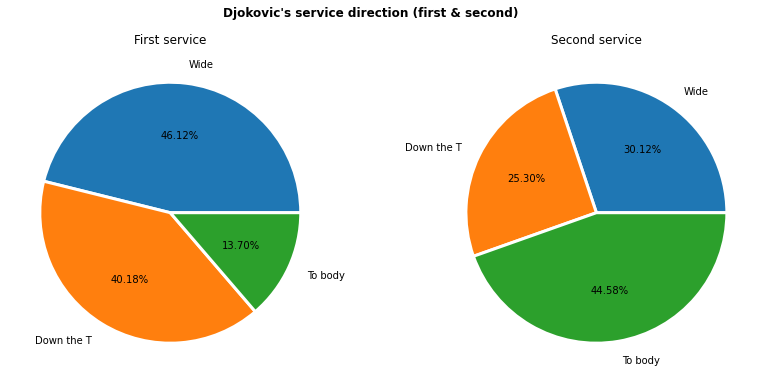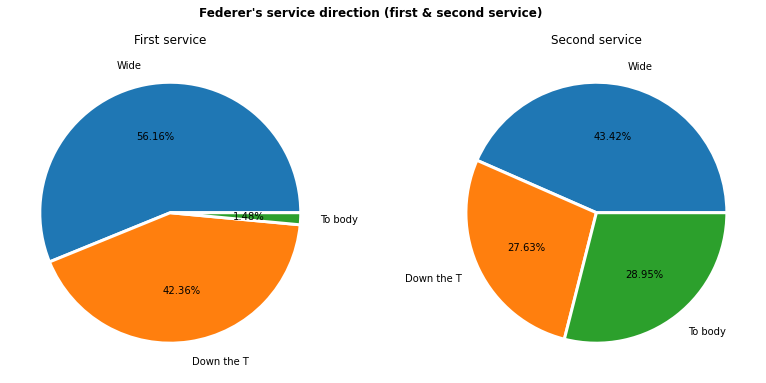Federer had only 1.48% of his first services of the opponent's body. In the second service, both players had many more services to body (maybe avoiding mistakes).

### Aces direction¶

In :
directions = ['wide','down the T','to body']
values1 = []
values2 = []
for direction in directions:
# First player data
values1.append(fs_df1.loc[direction, 'ace'] + ss_df1.loc[direction, 'ace'])
values2.append(fs_df2.loc[direction, 'ace'] + ss_df2.loc[direction, 'ace'])
values = [values1, values2]

positions = np.arange(3)

# Aces types
plt.figure(figsize=(12,6))
plt.bar(positions-0.1, values, width=0.2, color='g', align='center')
plt.bar(positions+0.1, values, width=0.2, color='y', align='center')
plt.ylabel('Number of aces')
plt.xticks(positions, directions)
plt.title('Aces by direction')
plt.legend(player_name)
plt.grid('minor')
plt.show()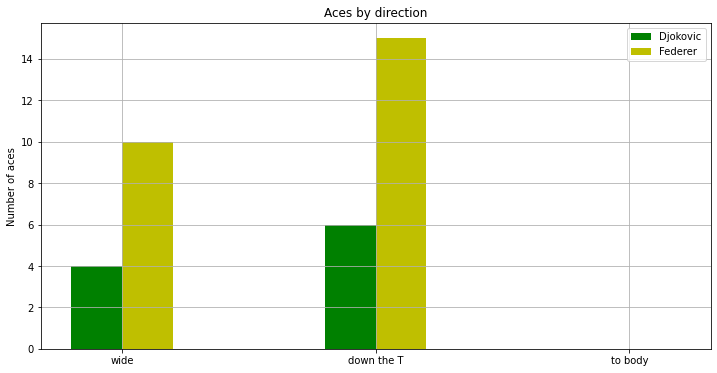Although most of the services for both players was wide, down the T was the direction in which both players had more aces. It was fair to assume that there was no aces directed to the body, but it is not bad to check, just to be sure :).

### Fault direction¶

In :
faults = ['fault (long)','fault (wide)','fault (net)','fault (wide and long)']
values1 = []
values2 = []
for fault in faults:
values1.append(fs_df1[fault].to_numpy().sum() + ss_df1[fault].to_numpy().sum())
values2.append(fs_df2[fault].to_numpy().sum() + ss_df2[fault].to_numpy().sum())
values = [values1, values2]

fig, axes = plt.subplots(nrows=1, ncols=2, figsize=(14,6), facecolor='white')
ax = axes.ravel()
plt.suptitle('Fault direction', fontweight='bold')
for i in range(2):
ax[i].pie(values[i],
labels=faults,
labeldistance=1.15,
wedgeprops = { 'linewidth' : 3, 'edgecolor' : 'white' },
autopct = '%.2f%%')
ax[i].set_title(player_name[i]+'\'s service')
plt.show()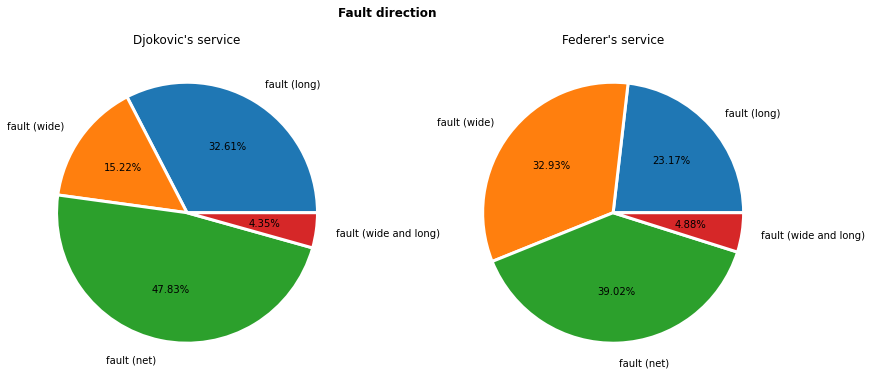The most common service fault is definitely net, but having in mind that most of the services were wide, Đoković's wide service errors are quite small and were made twice less frequently than Federer's faults.

## Service outcome¶

In :
names = ['ace', 'service winner', 'fault (long)', 'fault (wide)', 'fault (net)', 'fault (wide and long)', 'regular']
values1 = []
values2 = []
for name in names:
values1.append(fs_df1[name].to_numpy().sum() + ss_df1[name].to_numpy().sum())
values2.append(fs_df2[name].to_numpy().sum() + ss_df2[name].to_numpy().sum())
values = [values1, values2]

fig, axes = plt.subplots(nrows=1, ncols=2, figsize=(14,6), facecolor='white')
ax = axes.ravel()
plt.suptitle('Service outcome', fontweight='bold')
for i in range(2):
ax[i].pie(values[i],
labels=names,
labeldistance=1.15,
wedgeprops = { 'linewidth' : 3, 'edgecolor' : 'white' },
autopct = '%.2f%%')
ax[i].set_title(player_name[i]+'\'s service')
plt.show()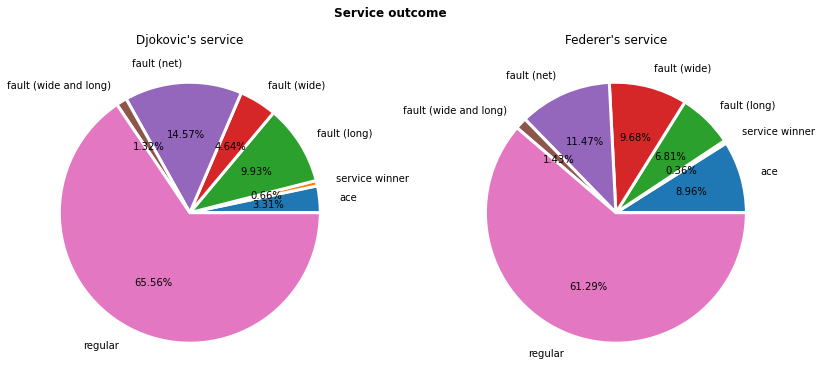From the pie chart above, we can maybe conclude that Federer's service is a bit more attacking with a significantly larger number of aces, but also with the largest percentage of errors made during the match.

# Drop shot¶

In :
drop_shots = [df.loc[df["drop_shot"]==1].shape, df.loc[df["drop_shot"]==2].shape]
position = 0

# Drop shots visualization
plt.figure(figsize=(12,6))
plt.bar(position-0.5, drop_shots, width=1, color='b', align='center')
plt.bar(position+0.5, drop_shots, width=1, color='y', align='center')
plt.ylabel('Number of drop shots')
plt.xticks([-0.5, 0.5], (player_name, player_name))
plt.xlim([-5,5])
plt.title('Number of drop shots per player')
plt.legend(player_name)
plt.grid(which='major', axis='y')
plt.show()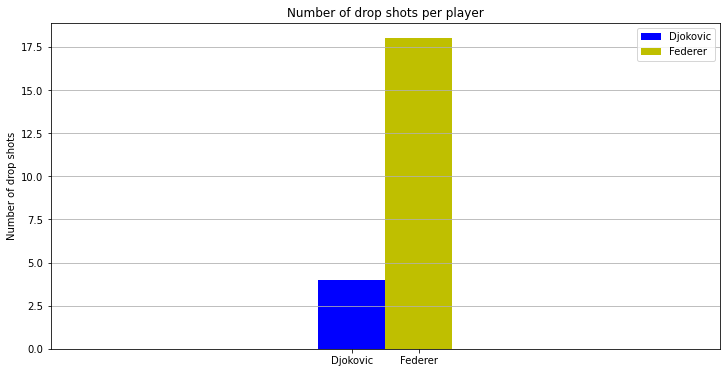As we analyse just one match, it cannot be said if Đoković is not a drop shot player or a drop shot is a way to go for Federer, but difference in playing tactics is obvious in this one.

# Lobs¶

In :
lobs = [df.loc[df["lob"]==1].shape, df.loc[df["lob"]==2].shape]
position = 0

# Lobs visualization
plt.figure(figsize=(12,6))
plt.bar(position-0.5, lobs, width=1, color='g', align='center')
plt.bar(position+0.5, lobs, width=1, color='m', align='center')
plt.ylabel('Number of lobs')
plt.xticks([-0.5, 0.5], (player_name, player_name))
plt.xlim([-5,5])
plt.title('Number of lobs per player')
plt.legend(player_name)
plt.grid('major', axis='y')
plt.show()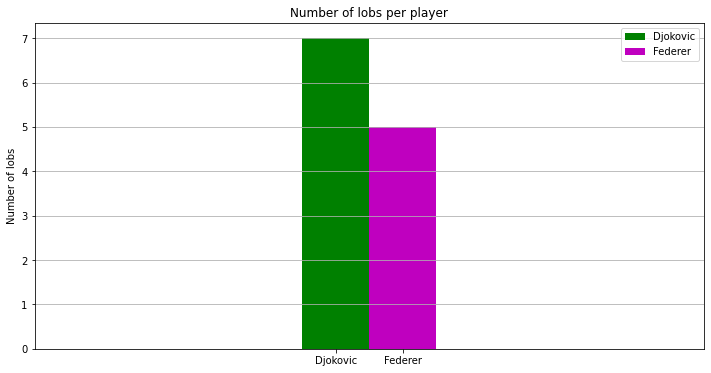Đoković had a few lobs more and much less drop shots to draw his opponent near net than Federer.

# Stroke analysis¶

Much more details are hidden in point description than just a serve. Most important among them are strokes. In further analysis, it is pointed out how strokes, such as forehand and backhand, differ for each player.

In :
player1_on_service = df.loc[df["server"] == player_name.lower()]

# Forehands
forehand = [{},{}]
# Backhands
backhand = [{},{}]

for player in range(2):

# Extract data for current server
player_on_service = df.loc[df["server"] == player_name[player].lower()]

# If first player has service then later on at first stroke itr%2 = 0
itr_init = 1 + player

# Going through every point description
for i in range(player_on_service.shape):

point_desc = player_on_service['point_description'].iloc[i].split(';')

# Iterator helps detecting current player
itr = itr_init

for desc in point_desc:
itr += 1

# Remove phrase 'return' if exists
desc = desc.replace('return ','')

# Check if forehand is played
if('forehand' in desc):
# Remove rally length
stroke_desc = desc.split('.')

# Remove 'forehand ' from description
stroke_desc = stroke_desc.replace(' forehand ','')

# Check for winner/error
if(len(stroke_desc.split(','))==2):
# Stroke type
stroke_type = stroke_desc.split(',')
# Finish type
point_end = stroke_desc.split(',')

# Check for winner
if('winner' in point_end):
# Check if key exists
if('winner' in forehand[itr%2].keys()):
forehand[itr%2]['winner'] += 1
else:
forehand[itr%2]['winner'] = 1

# Check for error
elif('error' in point_end):
# Error description
error_type = point_end[1:]

# Check if key exists
if(error_type in forehand[itr%2].keys()):
forehand[itr%2][error_type] += 1
else:
forehand[itr%2][error_type] = 1
else:
raise ValueError("New type came up on the end of the point: %s" %(point_end))
else:
if('rally' in forehand[itr%2].keys()):
forehand[itr%2]['rally'] += 1
else:
forehand[itr%2]['rally'] = 1

# Check if backhand is played
if('backhand' in desc):
# Remove rally length
stroke_desc = desc.split('.')

# Remove 'forehand ' from description
stroke_desc = stroke_desc.replace(' backhand ','')

# Check for winner/error
if(len(stroke_desc.split(','))==2):
# Stroke type
stroke_type = stroke_desc.split(',')
# Finish type
point_end = stroke_desc.split(',')

# Check for winner
if('winner' in point_end):
# Check if key exists
if('winner' in backhand[itr%2].keys()):
backhand[itr%2]['winner'] += 1
else:
backhand[itr%2]['winner'] = 1

# Check for error
elif('error' in point_end):
# Error description
error_type = point_end[1:]

# Check if key exists
if(error_type in backhand[itr%2].keys()):
backhand[itr%2][error_type] += 1
else:
backhand[itr%2][error_type] = 1
else:
raise ValueError("New type came up on the end of the point: %s" %(point_end))
else:
if('rally' in backhand[itr%2].keys()):
backhand[itr%2]['rally'] += 1
else:
backhand[itr%2]['rally'] = 1



## Strokes in general¶

In :
# Strokes in general
for i in range(2):
fig, axes = plt.subplots(nrows=1, ncols=2, figsize=(14,6), facecolor='white')
ax = axes.ravel()
plt.suptitle(player_name[i]+'\'s strokes', fontweight='bold')
values = [list(forehand[i].values()), list(backhand[i].values())]
labels = [list(forehand[i].keys()), list(backhand[i].keys())]
titles = ['Forehand', 'Backhand']
for j in range(2):
ax[j].pie(values[j],
labels=labels[j],
labeldistance=1.15,
wedgeprops = { 'linewidth' : 3, 'edgecolor' : 'white' },
autopct = '%.2f%%')
ax[j].set_title(titles[j])
plt.show()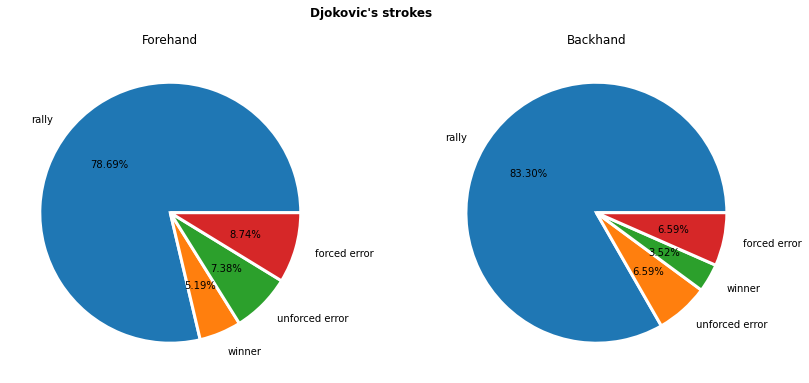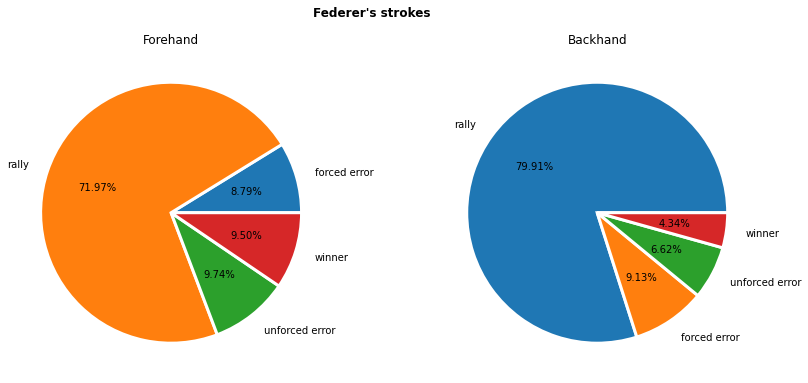Federer's forehand has the highest error rate and the greatest percentage of winners, while Đoković's backhand has the smallest percentage of errors and winner, making them the best attacking and the most stable strokes of these two players.

## Number of stroke types¶

In :
positions = np.array([-.5, .5])
winner = [forehand[i]['winner']+backhand[i]['winner'] for i in range(2)]
forced = [forehand[i]['forced error']+backhand[i]['forced error'] for i in range(2)]
unforced = [forehand[i]['unforced error']+backhand[i]['unforced error'] for i in range(2)]
rally = [forehand[i]['rally']+backhand[i]['rally'] for i in range(2)]

# Aces per sets
plt.figure(figsize=(12,6))
plt.bar(positions-0.2, rally, width=0.2, color='c', align='center')
plt.bar(positions, winner, width=0.2, color='y', align='center')
plt.bar(positions+0.2, forced, width=0.2, color='g', align='center')
plt.bar(positions+0.2, unforced, bottom=forced, width=0.2, color='m', align='center')
plt.ylabel('Number of strokes')
plt.xticks(positions, player_name)
plt.xlabel('Player')
plt.title('Stroke types per player')
plt.legend(['Rally', 'Winner', 'Forced error','Unforced error'])
plt.grid(which='major', axis='y')
plt.show()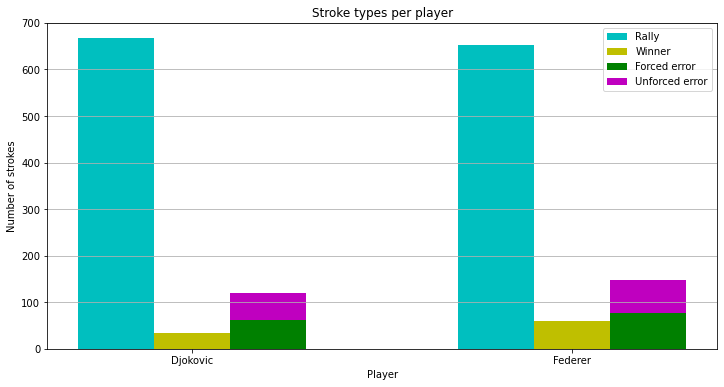Looking at the number of strokes (not just a relation in between stroke), Federer's strokes are more likely to end up as winners or errors then Đoković's strokes.

## Stroke errors¶

In :
labels = ['Forehand unforced error','Forehand forced error',
'Backhand unforced error','Backhand forced error']
values = [[forehand['unforced error'], forehand['forced error'],
backhand['unforced error'], backhand['forced error']],
[forehand['unforced error'], forehand['forced error'],
backhand['unforced error'], backhand['forced error']]]

fig, axes = plt.subplots(nrows=1, ncols=2, figsize=(14,6), facecolor='white')
ax = axes.ravel()
plt.suptitle('Stroke error', fontweight='bold')
for j in range(2):
ax[j].pie(values[j],
labels=labels,
labeldistance=1.01,
wedgeprops = { 'linewidth' : 3, 'edgecolor' : 'white' },
autopct = '%.2f%%')
ax[j].set_title(player_name[j])
plt.show()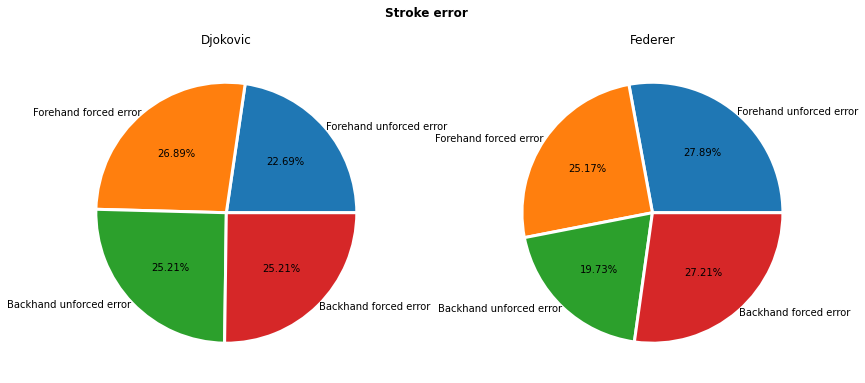Number of forced and unforced errors can be compared also as an overall stroke error analysis. Federer's unforced backhand error is the rarest mistake on the match and his his unforced forehand error the most common one. Đoković's errors, on the other hand, are quite balanced.

# Point ending¶

In :
labels = ['Error by opponent', 'Winner', 'Ace', 'Double fault']
values = [[],[]]
# Points won by first player
values.append(df.loc[df['error']==2].shape)
values.append(df.loc[df['winner']==1].shape)
values.append(df.loc[df['ace']==1].shape)
values.append(df.loc[df['double_fault']==2].shape)
# Points won by second player
values.append(df.loc[df['error']==1].shape)
values.append(df.loc[df['winner']==2].shape)
values.append(df.loc[df['ace']==2].shape)
values.append(df.loc[df['double_fault']==1].shape)

fig, axes = plt.subplots(nrows=1, ncols=2, figsize=(14,6), facecolor='white')
ax = axes.ravel()
plt.suptitle('Point ending', fontweight='bold')
for j in range(2):
ax[j].pie(values[j],
labels=labels,
labeldistance=1.15,
wedgeprops = { 'linewidth' : 3, 'edgecolor' : 'white' },
autopct = '%.2f%%')
ax[j].set_title(player_name[j]+'\'s winning points')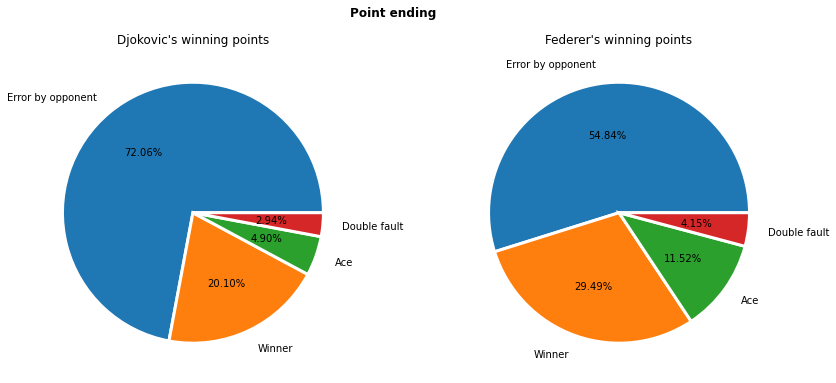Analysis started with points beginning (service), going through points "body" (strokes) and the last one is how point ends. Đoković relies on an opponent's mistake with 75% of his winning points and afterwards on winners that are one fifth of all points he has won. Federer on the other hand didn't count on opponent's preparedness or endurance and Đoković's mistakes gave him around 60% of all of his points, with his winners around 30% and aces over 11%. This may not seem much by looking at the chart above, but Đoković had only 25% won "by his own" comparing to Federer's 41%.

# Comparing point's winner and point's rally length¶

In :
points_won = [[],[]]
max_rally = np.amax(df['rally_length'].to_numpy())+1
for i in range(2, max_rally):
for j in range(2):
points_won[j].append(df.loc[(df['rally_length']==i) & (df['pt_won_by']==(j+1))].shape)

positions = np.arange(2, max_rally)

plt.figure(figsize=(12,6))
plt.bar(positions-0.1, points_won, width=0.2, color='g', align='center')
plt.bar(positions+0.1, points_won, width=0.2, color='y', align='center')
plt.ylabel('Number of points won')
plt.xlabel('Rally length')
plt.xticks(positions, positions)
plt.title('Winning points by rally length')
plt.legend(player_name)
plt.grid(which='major', axis='y')
plt.show()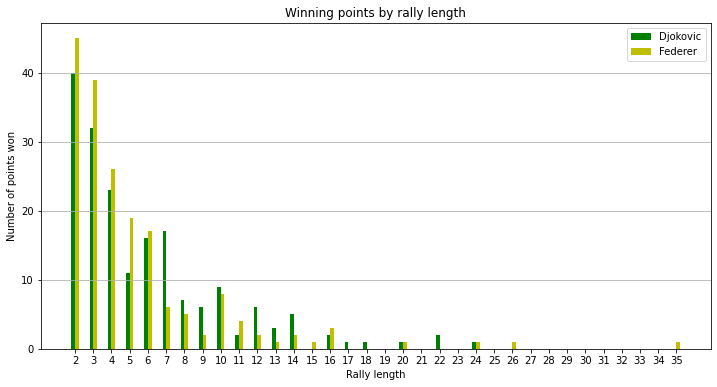For short rallies, Federer has a better score of winning the point, but when it comes to medium and long rallies, Đoković have performed better.

# Distribution of points won with respect to point duration¶

In :
duration = []
for i in range(1,3):
duration.append(df.loc[df['pt_won_by'] == i]['point_duration'])

plt.figure(figsize=(12,8))
sn.kdeplot(duration, label=player_name)
sn.kdeplot(duration, label=player_name)
plt.ylabel('Probability  of winning a point')
plt.xlabel('Point duration')
plt.xlim([0,np.amax(df['point_duration'])])
plt.title('Distribution of points won with respect to point duration')
plt.grid('minor')
plt.show()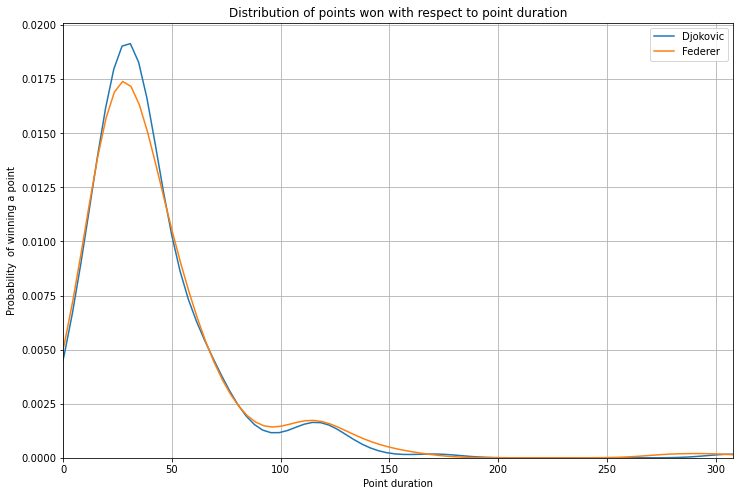Both players have had a similar distribution of points won depending on point duration. Some rough estimate should suggest that points up to 60 seconds are the one that Đoković can turn in his favour and longer points are more Federer "cup of tea".

# Points duration¶

In :
match_dur = df['vid_second'].iloc[-1] - df['vid_second'].iloc + df['point_duration'].iloc[-1]
h = match_dur//3600
m = (match_dur%3600)//60
s = (match_dur%3600)%60
print('Match duration is: %dh:%dm:%ds' %(h,m,s))

# Points duration
fig, axes = plt.subplots(ncols=2, figsize=(18,6))
ax = axes.ravel()
plt.suptitle("Point duration")

# Show timestamps of
ax.scatter(df['vid_second'],df['point_duration'])
ax.set_xlabel("Video [s]")
ax.set_ylabel("Timestamp of the match [s]")
ax.set_title('Point duration during the match')
ax.grid('minor')

# Show boxchart of points duration
sn.boxplot(ax=ax, x=df['point_duration'])

plt.show()

Match duration is: 4h:56m:26s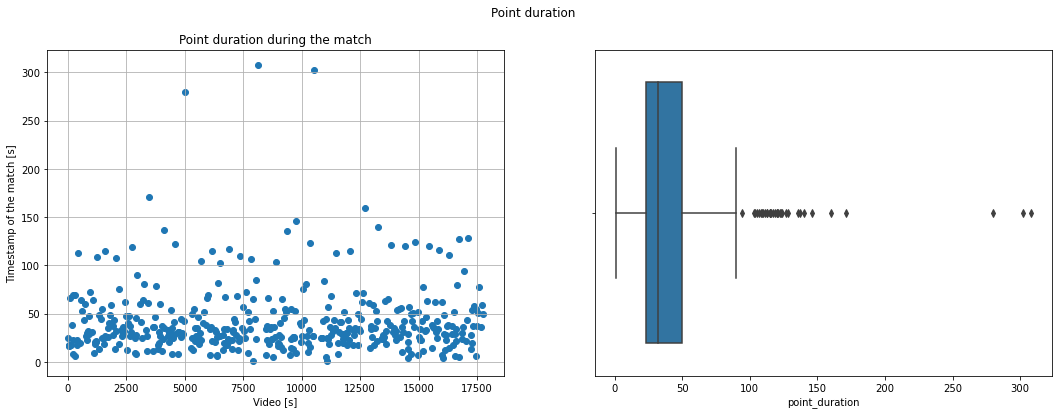Median of point duration is about 40 seconds with three longest points around 5 minute player in the middle of the game. One fourth of all points have ended up with less then 25 seconds and over one fourth of all points lasted over 50s. With match duration of nearly 5 hours without a doubt it is one of the longest, if not the longest, Wimbledon finals.

## Rally length¶

In :
# Points duration
fig, axes = plt.subplots(ncols=2, figsize=(18,6))
ax = axes.ravel()
plt.suptitle("Rally length")

# Show timestamps of
ax.scatter(df['vid_second'],df['rally_length'])
ax.set_xlabel("Timestamp of the match [s]")
ax.set_ylabel("Rally length")
ax.set_title('Rally length during the match')
ax.grid('minor')

# Show boxchart of points duration
sn.boxplot(ax=ax, x=df['rally_length'])

plt.show()